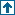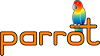parrotcode: Calculate prime numbers < 50000 Contents | Examples

# NAMEexamples/benchmarks/primes.c - Calculate prime numbers < 50000

# SYNOPSIS```    % make examples/benchmarks/primes
% time examples/benchmarks/primes```

# DESCRIPTIONCalculates all the prime numbers up to 50000 and prints out the number of primes and the last one found.

# SEE ALSO